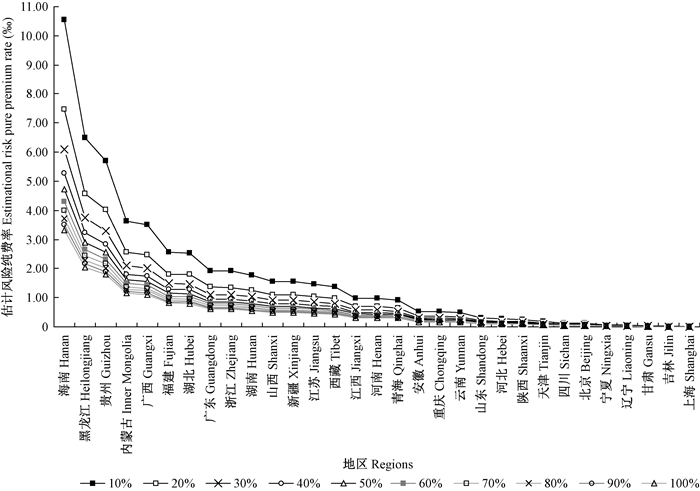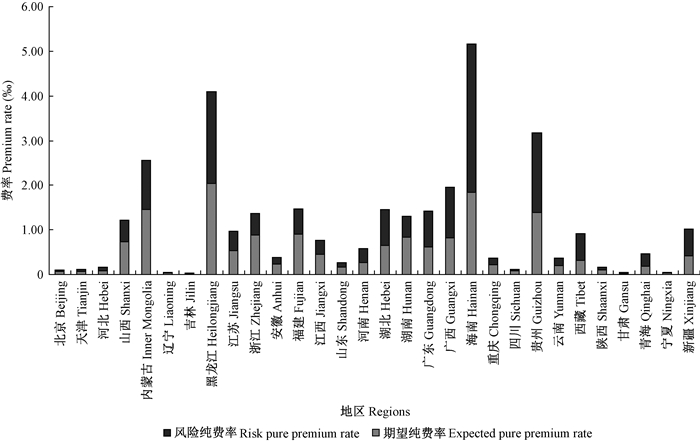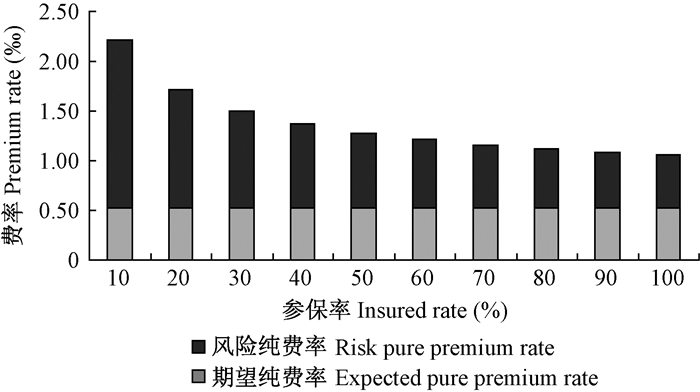林业科学2016, Vol. 52Issue (7): 129-137PDF
DOI: 10.11707/j.1001-7488.20160716
0

#### 文章信息

Zhang Decheng, Chen Shaozhi, Bai Dongyan

Model and Empirical Analysis on Forest Fire Insurance Pure Premium Rate Making

Scientia Silvae Sinicae, 2016, 52(7): 129-137.
DOI: 10.11707/j.1001-7488.20160716

### 作者相关文章

1. 中国林业科学研究院林业科技信息研究所 北京 100091;
2. 北京市经济管理学校财会金融系 北京 100142

Model and Empirical Analysis on Forest Fire Insurance Pure Premium Rate Making
Zhang Decheng1, Chen Shaozhi1, Bai Dongyan2
1. Research Institute of Forestry Policy and Information, CAF Beijing 100091 ;
2. Department of Accounting and Finance, Beijing Economic and Management College Beijing 100142
Key words: forest insurance     pure premium rate     rate making     forest fire

Holecy等(20032006)构建的纯费率模型纳入了森林火灾发生概率、森林火灾年际差异以及参保面积等因素，但该模型在费率单位、动态保额等方面与我国现行森林保险制度存在一定差异，不能直接用于我国森林保险费率厘定，需对该模型进行适当转换和改进。本文基于Holecy等(20032006)的研究，简化和改进森林火灾保险纯费率厘定模型，运用简化和改进后的模型估计2014年我国各地区不同参保率下的森林火灾保险纯费率水平，对比分析19个试点地区森林火灾保险估计纯费率和实际纯费率，以检验估计结果的准确性，并对模型应用提出建议。

1 模型和方法 1.1 模型设定 1.1.1 Holecy模型

Gm(t)为投保面积为m、林龄为t的森林火灾保险纯费率(€·a-1hm2)，N(t)为期望纯费率(€·a-1hm-2)，Rm(t)为风险纯费率(€·a-1hm-2)。Gm(t)的计算公式为：

 ${G_m}\left( t \right) = N\left( t \right) + {R_m}\left( t \right) \circ$ (1)

 $N\left( t \right) = H\left( t \right) \cdot p\left( t \right) \circ$ (2)

p(t)的计算公式为：

 $p\left( t \right) = u \cdot \Delta F\left( t \right) \cdot \hat p \circ$ (3)

 $\hat p = \frac{h}{H}$ (4)

 $F\left( t \right) = 1 - {{\rm{e}}^{ - c{t^r}}} \circ$ (5)

 ${R_m}\left( t \right) = H\left( t \right) \cdot u \cdot \Delta F\left( t \right) \cdot {S_m} \cdot {z_{\alpha /2}} \circ$ (6)

 ${S_m} = \sqrt {\frac{{\hat p\left( {1 - \hat p} \right)}}{m}} \circ$ (7)

 $\begin{array}{l} {G_m}\left( t \right) = H\left( t \right) \cdot p\left( t \right) + H\left( t \right) \cdot u \cdot \Delta F\left( t \right) \cdot \\ \sqrt {\frac{{\hat p\left( {1 - \hat p} \right)}}{m}} \cdot {Z_{\alpha /2}} \circ \end{array}$ (8)
1.1.2 对Holey模型的简化和改进

1) 转换为固定保额下的总体森林火灾保险费率模型。由于只考虑森林总体的火灾发生概率，不考虑林龄差异，因此式(3)中，p(t)=${\hat p}$ΔF(t)=1。采用固定保额制度，因此式(2)和式(6)中，保额H(t)为常数，设H(t)=n，这样式(8)可写成如下形式：

 ${G_m}\left( t \right) = n \cdot \hat p + n \cdot {S_m} \cdot {Z_{\alpha /2}} \circ$ (9)

2) 费率单位转换为千分率。设投保森林面积为m的火灾保险纯费率为Pm(‰)，期望纯费率为 EPm(‰)，风险纯费率为RPm(‰)，由于千分率费率乘以保额就是以实际货币量计量的费率，即，Gm(t)=Pm·nN(t)=EPm·n，Rm(t)=RPm·n，代入式(1)中，两边除以n，得到：

 ${P_m} = {\rm{E}}{{\rm{P}}_m} + {\rm{R}}{{\rm{P}}_m} \circ$ (10)

N(t)=EPm·n代入式(2)得到：

 ${\rm{E}}{{\rm{P}}_m} = \hat p \circ$ (11)

Rm(t)=RPm·n代入式(6)得到：

 ${\rm{R}}{{\rm{P}}_m} = {S_m} \cdot Z \circ$ (12)

 ${P_m} = \hat p + {S_m} \cdot {Z_{\alpha /2}} \circ$ (13)

3) 以参保率代替参保面积变量。设M为区域森林总面积，参保率为β，则对于参保面积为m=βM的森林，可以计算不同参保率下的标准误差值SβM

 ${S_m} = {S_{\beta M}} = \sqrt {\frac{{\hat p\left( {1 - \hat p} \right)}}{{\beta M}}} = \frac{1}{{\sqrt \beta }} \cdot \sqrt {\frac{{\hat p\left( {1 - \hat p} \right)}}{M}} = \frac{{{S_M}}}{{\sqrt \beta }} \circ$ (14)

 ${S_M} = \sqrt {\frac{{\sum\limits_{i = 1}^T {{{\left( {\hat p - {p_i}} \right)}^2}} }}{{T - 1}}} \circ$ (15)

 ${\rm{R}}{{\rm{P}}_m} = {\rm{R}}{{\rm{P}}_{\beta M}} = \frac{{{S_M}}}{{\sqrt \beta }} \cdot {Z_{\alpha /2}}^\circ$ (16)

 ${p_m} = {p_{\beta M}} = \hat p + \frac{{{S_M}}}{{\sqrt \beta }} \cdot {Z_{\alpha /2}} \circ$ (17)
1.1.3 估计费率与实际费率对比分析模型

 $C_{\beta M}^ * = \frac{{P_{\beta M}^ * \cdot c}}{{G \cdot c}} = \frac{{P_{\beta M}^ * }}{G}^\circ$ (18)

 $P_{\beta M}^ * = C_{\beta M}^ * \cdot G^\circ$ (19)
1.2 数据收集及统计分析方法

1949年以来，我国森林防火经历了5个阶段(施一凡，2012)。1990年以后，森林火灾管理手段不断创新，林火发生次数和受灾面积大幅减少(蔡亚林，2007)。为估计2014年森林火灾保险纯费率，本文采用1991—2013年的森林面积、森林火灾受害面积数据，数据来源于 《中国林业统计年鉴》，对第4~8次森林清查各地区森林面积数据进行线性插值，以每次清查末年作为清查结果数据年，以此为基准向前插值计算各地区历年森林面积。

2014年各地区森林保险毛费率、赔付率、参保森林面积数据来源于国家林业局发展规划与资金管理司提供的2014年各试点地区森林火灾保险统计资料，该资料中有19个试点地区数据，依据式(19)求出实际纯费率。对2014年19个地区的估计纯费率和实际纯费率进行对比分析，方法采取配对样本T检验(paired samples T test)，数据分析软件采用SPSS13.0。

2 结果与分析 2.1 各地区森林火灾保险的期望纯费率

1991—2013年各地区森林火灾发生概率在0~37.15‰之间(表 1)，最大值为海南(37.15‰)，其次为贵州(20.35‰)，第三为黑龙江(17.38‰)，其他地区近23年来发生最大森林火灾的年份，森林火灾受害面积均不超过该地区森林面积的10.00‰，多数都在5.00‰以下。

2014年各地区森林火灾保险期望纯费率(EPM=${\hat p}$)在0~2.04‰之间，绝大多数地区都在1.00‰以下(表 2)，上海、吉林等9个地区的期望纯费率在0.10‰以下； 陕西、山东等18个地区在0.10‰~1.00‰之间，只有黑龙江、贵州、内蒙古、海南超过了1.00‰，其中黑龙江省最高，达到2.04‰。

2.2 各地区森林火灾保险的风险纯费率

2014年各地区森林火灾期望概率的标准误差(SM)在0~0.29‰之间，森林火灾发生概率的标准差值均超过了均值(表 1)，表明年际间森林火灾发生面积的差异性明显。由于标准差值较大，31个地区中，森林火灾发生概率的一致性双尾检验值大于0.05的仅有11个，表示大多数地区的森林火灾发生概率均值不能代表样本值的数据波动，因此在厘定森林火灾保险费率时需要增加风险纯费率数据来反映年度差异。图 1 2014年各地区不同投保比例下的森林火灾保险估计风险纯费率(RP) Fig.1 RP of forest fire insurance under different insured rate by regions in 2014
2.3 各地区森林火灾保险的纯费率图 2 2014年各地区森林火灾保险参保率为100%状况下的期望纯费率(EPM)与风险纯费率(RPβM) Fig.2 EPM and RPβM of forest fire insurance under 100%insured rate by regions in 2014图 3 2014年不同森林火灾保险参保率下全国平均风险纯费率和期望纯费率 Fig.3 Average RPβM and EPβM of forest fire insurance under different insured rate in 2014
2.4 估计纯费率与实际纯费率的对比分析4 结论

2014年我国19个森林保险试点地区的森林火灾保险估计纯费率阈值在0.05‰~7.20‰之间，实际纯费率在0.07‰~3.25‰之间，经均值比较，估计纯费率略大于实际纯费率，根据配对样本T检验，估计纯费率与实际纯费率无差异，是符合实际情况的合理取值。本模型充分考虑了森林火灾的确定因素和不确定性因素，厘定费率适用于我国现行森林保险制度，符合我国森林火灾保险现状，模型估计结果准确可靠。

5 讨论和建议

 [] 白冬艳, 翟印礼, 陈绍志, 等. 2013. 基于自回归移动平均(ARMA)模型的森林火灾预测. 林业实用技术 , 21 (6) : 72–73. ( Bai D Y, Zhai Y L, Chen S Z, et al.2013. Forest fire prediction based on auto regressive moving average (ARMA) model. Practical Forestry Technology , 21 (6) : 72–73. [in Chinese] ) (0) [] 蔡亚林. 2007. 在责任与挑战中稳步前进——近二十年我国森林防火工作综述. 经济 (7) : 110–111. ( Cai Y L.2007. The responsibilities and challenges of steady progress in nearly twenty years of China's forest fire prevention work summary. Economy (7) : 110–111. [in Chinese] ) (0) [] 黄向阳, 金阳, 肖宇谷, 等. 2009. 精算中常用的统计模型. 北京: 中国人民大学出版社 . ( Huang X Y, Jin Y, Xiao Y G, et al. 2009. Statistical models used in actuarial science. Beijing: Renmin University of China Press . [in Chinese] ) [] 黄祖梅. 2014. 一个森林保险模型及最优免赔额的确定. 林业经济问题 , 34 (3) : 200–204. ( Huang Z M.2014. A forest insurance model and the determination of perfect deductible. Issues of Forestry Economics , 34 (3) : 200–204. [in Chinese] ) (0) [] 姜昕, 罗添元. 2014. 森林保险的费率厘定与业务实施要点分析. 绿色科技 (12) : 98. ( Jiang X, Luo T Y.2014. Study on the rate making of forest insurance and key points in its implementation. Journal of Green Science and Technology (12) : 98. [in Chinese] ) (0) [] 冷慧卿, 王珺. 2011. 我国森林保险费率的区域差异化——省级层面的森林火灾实证研究. 管理世界 (11) : 49–54. ( Leng H Q, Wang J.2011. Regional differences of forest insurance rates in China:an empirical study on the forest fire at the provincial level. Management World (11) : 49–54. [in Chinese] ) (0) [] 潘家坪. 1999. 森林保险中合理确定保险费率的探讨. 林业资源管理 (5) : 5–8. ( Pan J P.1999. Discussion on reasonable determination of insurance premium rate in forest insurance. Forest Resources Management (5) : 5–8. [in Chinese] ) (0) [] 王珺, 冷慧卿. 2011. 中央财政森林保险保费补贴六省试点调研报告. 保险研究 (2) : 48–56. ( Wang J, Leng H Q.2011. The research report on the pilot program of fiscal subsidy for forest insurance premium in six provinces. Insurance Studies (2) : 48–56. [in Chinese] ) (0) [] 王晓军. 2010. 保险精算原理与实务. 北京: 中国人民大学出版社 . ( Wang X J. 2010. actuarial principles and practice. Beijing: Renmin University of China Press . [in Chinese] ) [] 张德成, 陈绍志, 白冬艳. 2015. 森林保险毛费率及保额计算模型研究——基于纯保费法. 林业经济 (7) : 25–28,33. ( Zhang D C, Chen S Z, Bai D Y.2015. A forest insurance gross premium rate and sum insured calculation model:based on pure premium method. Forestry Economics (7) : 25–28,33. [in Chinese] ) (0) [] 施一凡. 2012. 我国森林防火历史沿革从一片空白到科学规范. 中国绿色时报 : 10–15. ( Shi Y F.2012. History of forest fire prevention in China from a blank to scientific and standardized. China Green Times : 10–15. [in Chinese] ) (0) [] Bernard L W, Harrison C M, Markham J J. 1997. Insurance operations. Malvern:American Institute for Chartered Property Casualty Underwriters. [] Cipriano P A, Almeida R N.2013. Forest property insurance:an application to Portuguese woodlands. International Journal of sustainable society (3) : 284–295. (0) [] Davis E W, Pointon J. 1994. Finance and the firm:an introduction to corporate finance. New York: Oxford Univ Press, USA . [] Ehrlich I, Becker G.1972. Market insurance, self-insurance and self-protection. Journal of Political Economy , 80 (4) : 623–648. (0) [] Gadow K V.2000. Evaluating risk in forest planning models. Silva Fennica , 34 (2) : 181–191. (0) [] Holecy J, Skvarenina J, Tucek J, et al. 2003. Fire risk insurance model for forest stands growing in the area of Slovak Paradise. Athens:Institute of Mediterranean Forest Ecosystems and Forest Products Technology. [] Holecy J, Hanewinkel M.2006. A forest management risk insurance model and its application to coniferous stands in southwest Germany. General Information , 8 (2) : 161–174. (0) [] Marielle B, Couture S, Garcia S. 2011. Insurance demand against forest fire risk:empirical analysis on french private forest owners. New York: Social Science Electronic Publishing .